# RS Aggarwal Class 9 Solutions Chapter 5 - Congruence And Inequalities In Triangle Ex 5A (5.1)

## RS Aggarwal Class 9 Chapter 5 – Congruence And Inequalities In Triangle Ex 5A (5.1) Solutions Free PDF

The RS Aggarwal Class 9 Solutions provided in our BYJU’S website are prepared by our experienced subject experts on the basis of the official latest syllabus approved by the CBSE. Students are advised to start their preparation by solving these solutions which will clear your concepts so that you can score good marks in your final exam. Refer to these solutions while solving the exercise questions from the textbook so that you can idea about the solving steps.

Students of Class 9 are advised to practice the RS Aggarwal Class 9 Solutions Chapter 5 – Congruence And Inequalities In Triangle Ex 5A (5.1) to score well in their exam as it covers the complete syllabus and gives ample number of question from each and every topic for students to practice.

## Download PDF of RS Aggarwal Class 9 Solutions Chapter 5 – Congruence And Inequalities In Triangle Ex 5A (5.1)

Question 1:

In the adjoining figure, PA $\perp$ AB, QB $\perp$ AB and PA = QB. If PQ intersects AB at O, show that O id the mid-point of AB as well as that of PQ.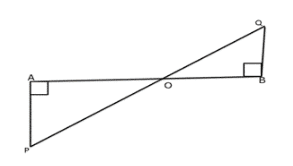Solution:

Given: PA $\perp$ AB, QB $\perp$ AB, and PA = QB

To prove: AO = OB and PO = OQ

Proof: In $\Delta$APO and $\Delta$BPO,

$\angle$PAO = $\angle$QBO = 90o [Given]

PA = QB [Given]

$\angle$PAO = $\angle$QBO [Since PA $\perp$ AB, and QB $\perp$ AB, PA || QB, and thus PQ is a transversal, therefore, alternate angles are equal]

So, by Angle-Side-Angle criterion of congruence, we have

$\Delta$APO $\cong$ $\Delta$BPO

$\Rightarrow$ AO = OB and PO = OQ [Since corresponding parts of congruent triangles are equal]

Thus, we have O is the midpoint of AB and PQ.

Question 2:

Let the line segments AB and CD intersect at O in such a way that OA = OD and OB = OC. Prove that AC = BD but AC may not be parallel to BD.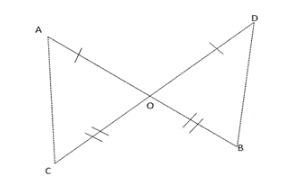Solution:

Given: Line segments AB and CD intersect at O such that OA = OD and OB = OC.

To prove: AC = BD

Proof: In $\Delta$AOC and $\Delta$BOD, we have

AO = OD [Given]

$\angle$AOC  = $\angle$BOD [Vertically opposite angles are equal]

OC = OB [Given]

So, by Side-Angle-Side criterion of congruence, we have,

$\Rightarrow$ AOC $\cong$ $\Delta$BOD

$\Rightarrow$ AC = BD [Since the corresponding parts of the congruent parts of the congruent triangles are equal]

$\Rightarrow$ $\angle$CAO = $\angle$BDO [by c.p.c.t]

Thus, we have, AC = BD

In case   $\angle$ODB = $\angle$OBD, then $\angle$CAO = $\angle$OBD which means alternate angles made by lines AB and BD with transversal AB are equal and then lines AC and BD will be parallel.

Question 3:

In the given figure, l||m and M is the mid-point of AB. Prove that M is also the mid-point of any line segment CD having its end points at l and m respectively.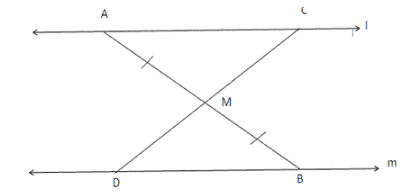Solution:

Given: Two lines l and m are parallel to each other. M is the midpoint of segment AB. The line segment CD meets AB at M.

To prove: M is the midpoint of CD, that is, CM = MD

Proof:

In $\Delta$AMC and $\Delta$BMD, we have

$\angle$MAC = $\angle$MBD [Since l and m are parallel, AB is the transversal, and thus, alternate angles are equal]

AM = MB [Given]

$\angle$AMC = $\angle$BMD [vertically opposite angles are equal]

So, by Angle-Side-Angle criterion of congruence, we have

$\Delta$AMC $\cong$ $\Delta$BMD

Therfore, by corresponding parts of the congruent triangles are equal, we have, CM = MD

Question 4:

In the given figure, AB = AC and OB = OC. Prove that $\angle$ABO = $\angle$ACO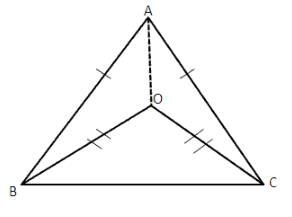Solution:

Given: AB = AC and O is an interior point of the triangle such that OB = OC

To prove: $\angle$ABO = $\angle$ACO

Construction: Join AO

Proof:

In $\Delta$AOB and $\Delta$AOC, we have

AB = AC [Given]

AO = AO [Common]

OB = OC [Given]

So, by Side-Side-Side criterion of congruence, we have,

$\Delta$ABO $\cong$ $\Delta$ACO

$\Rightarrow$ $\angle$ABO = $\angle$ACO [by corresponding parts of congruent triangles are equal]

Question 5:

In the given figure, ABC is a triangle in which AB = AC and D is a point AB. Through D, a line DE is drawn parallel to BC and meeting AC at E. Prove that AD = AE.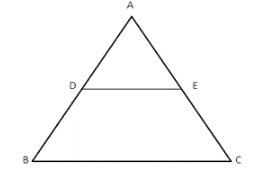Solution:

Given: A $\Delta$ABC in which;

AB = AC

and, DE|| BC

To prove: AD = AE

Proof:

Since DE || BC and AB is atransversal.

So, $\angle$ADE = $\angle$ABC —— (i) [These are corresponding angles]

Also DE || BC and AC is a transversal

So, $\angle$AED = $\angle$ACB —— (ii) [These are corresponding angles]

But, AB = AC [Given]

So, $\angle$ABC = ACB ——- (iii)

as opposite angles are also equal in case sides are equal

So from (i), (ii) and (iii) we have

$\angle$ADE = $\angle$AED

and in $\Delta$ADE, this implies that AD = AE.

Question 6:

In the adjoining figure, X and Y are respectively two points on equal sides AB and AC of $\Delta$ABC such that AX = AY. Prove that CX = BY.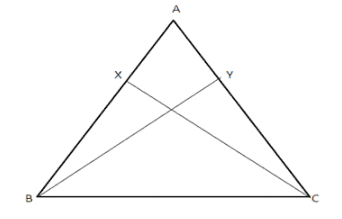Solution:

Given: AX = AY

To prove: CX = BY

Proof: In $\Delta$AXC and $\Delta$AYB, we have

AX = AY [Given]

$\angle$A = $\angle$A [Common angle]

AC = AB [Two sides are equal]

So, by Side-Angle-Side criterion of congruence, we have

$\Delta$AXC $\cong$ $\Delta$AYB

$\Rightarrow$ XC = YB [Since corresponding parts of congruent triangles are equal]

Question 7:

In the given figure, C is the mid-point of AB. If $\angle$DCA = $\angle$ECB and $\angle$DBC = $\angle$EAC, prove that DC = EC.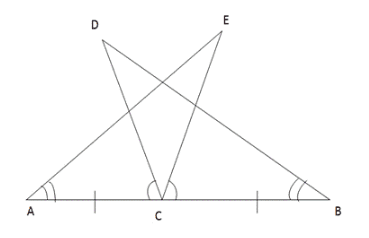Solution:

Given: C is the mid-point of a line segment AB, and D is point such that,

$\angle$DCA = $\angle$ECB

and $\angle$DBC = $\angle$EAC

To prove: DC = EC

Proof:

In $\Delta$ACE and $\Delta$DCB we have;

AC = BC [Given]

$\angle$EAC = $\angle$DBC [Given]

Also, $\angle$DCA = $\angle$CDB + $\angle$DBA because exterior $\angle$DCA in $\Delta$DCB is equal to sum of interior opposite angles.

Again in $\angle$ACE, we have

ext. $\angle$BCE = $\angle$CAE + $\angle$AEC

But, $\angle$DCA = $\angle$BCE [Given]

$\Rightarrow$ $\angle$CDB + $\angle$DBA = $\angle$CAE + $\angle$AEC

$\Rightarrow$ $\angle$CDB = $\angle$AEC [$\angle$DBA = $\angle$CAE (Given)]

Thus in $\Delta$ACE and $\Delta$DCB,

$\angle$EAC = $\angle$DBC

AC = BC

and, $\angle$AEC = $\angle$CDB

Thus by Angle-Side-Angle criterion of congruence, we have

$\Delta$ACE $\cong$ $\Delta$DCB

The corresponding parts of the congruent triangles are equal.

So, DC = CE [by c.c.p.c.t]

Question 8:

In the given figure, BA $\perp$ AC and DE $\perp$ EF such that BA = DE and BF = DC. Prove that AC = EF.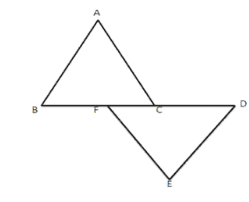Solution:

Given: AB $\perp$ AC and DE $\perp$ FE such that,

AB = DE and BF = CD

To prove: AC = EF

Proof:

In $\Delta$ABC, we have,

BC = BF + FC

and, in $\Delta$DEF

FD = FC + CD

But, BF = CD [Given]

So, BC = BF + FC

and, FD = FC + BF

$\Rightarrow$ BC = FD

So, in $\Delta$ABC and $\Delta$DEF, we have,

$\angle$BAC = $\angle$DEF = 90o [Given]

BC = FD [Proved above]

AB = DE [Given]

Thus, by Right angle-Hypotenuse-Side criterion of congruence, we have

$\Delta$ABC $\cong$ $\Delta$DEF

The corresponding parts of the congruent triangle are equal.

So, AC = EF [c.p.c.t]

AE = $\angle$BCD [Proved above]

Thus by Angle-Side-Angle criterion of congruence, we have

$\Delta$BCD $\cong$ $\Delta$BBAE

The corresponding parts of the congruent triangles are equal.

So, CD = AE [Proved]’

### Key Features of RS Aggarwal Class 9 Solutions Chapter 5 – Congruence And Inequalities In Triangle Ex 5A (5.1)

• The solutions are explained in a proper stepwise format.
• The solutions cover all the topics mentioned in the syllabus.
• It is a vital resource for students of Class 9 from exam view point.
• It improves academic performance and also boost students confidence.